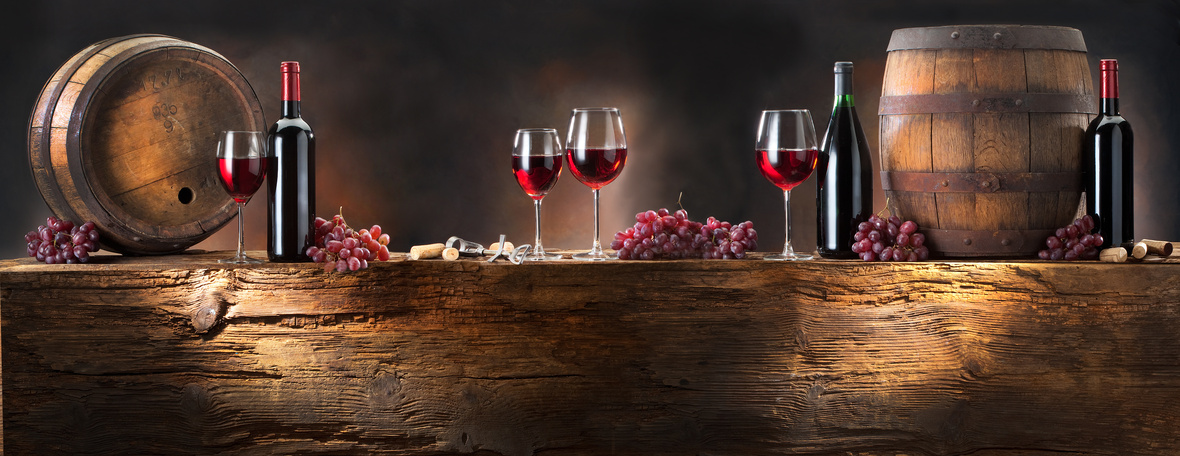Bourbon Month - No Party Like A Bourbon Party!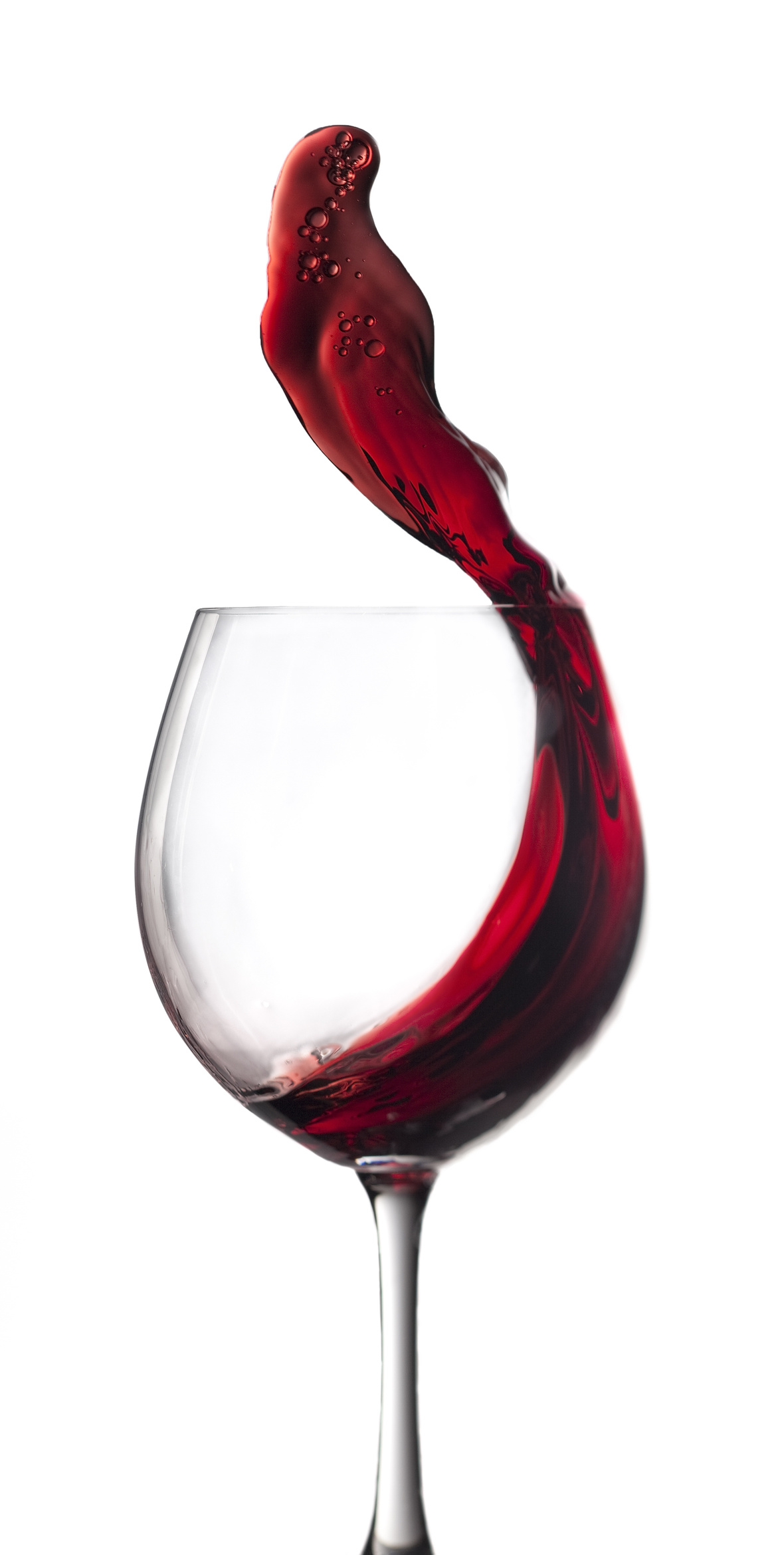Hi Wine & Spirits Lover,

The Shall We Wine team is excited to share our monthly newsletter. Our goal is to introduce you to our favorite selections, producers, distillers, varietals, regions and places to purchase and drink fabulous wine and spirits!

Cheers,

The Shall We Wine Team

 /* styles */

BOURBON LOVE! ♥To Bourbon, with love.

Bourbon is an exciting shift for many existing wine lovers. Not only does Bourbon tasting often mimic the comforts of the wine drinking process, there's the added fun of possible cocktails! In addition, wine drinkers might be interested in the growing number of Bourbon options finished in wine casks! Fascinating.

This is our ode to the beloved Bourbon. Over the years we've seen a major surge in Bourbon offerings and you'll find varied selections made all over the country.

 table div table+table+table+table+table+table+table div table{width:100%;padding:0}table div table+table+table+table+table+table+table div table img{width:96.23%;padding:0;float:none}table div table+table+table+table+table+table+table div table td{width:100%;padding:0 1.88% 18px}/* styles */SAVE THE DATE: BOURBON MONTH VIRTUAL EVENT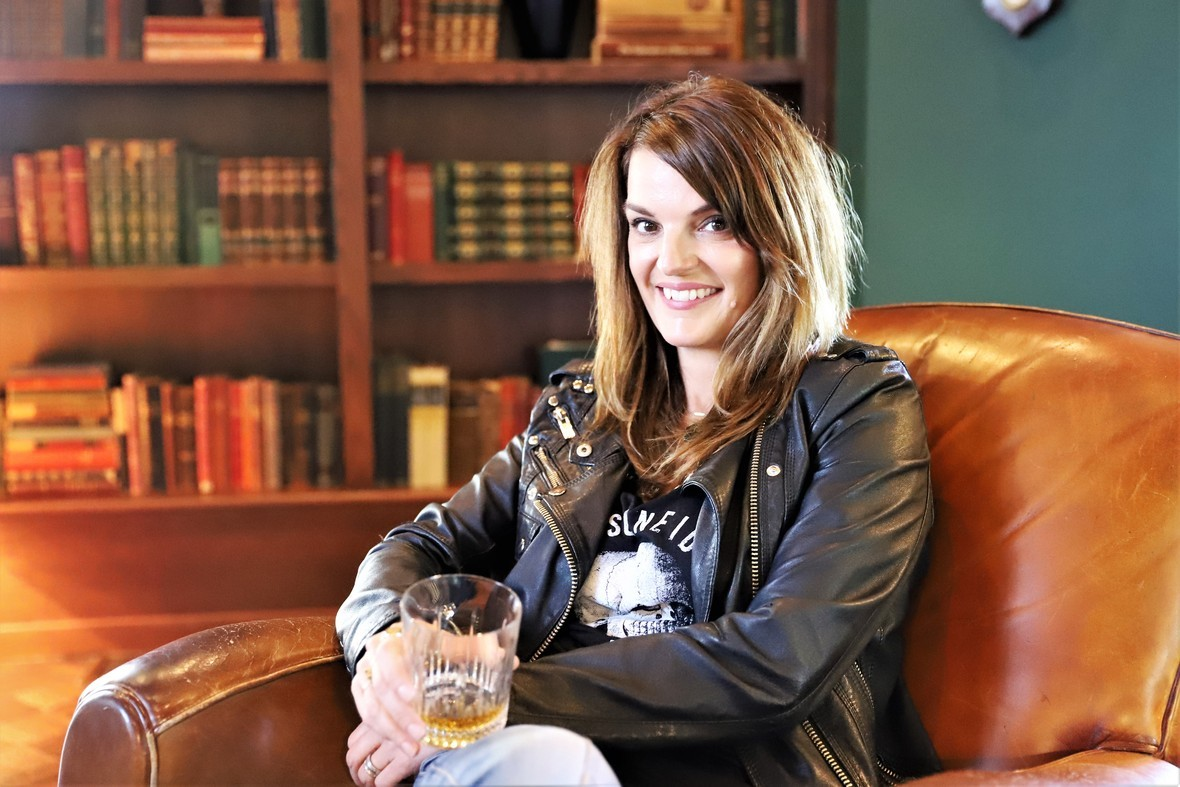Who: Heather Greene - CEO, PARTNER, MASTER BLENDER

World-renowned whiskey expert, author and master blender, Heather Greene, leads the Provision Spirits business with direct oversight of product development, including blending her namesake whiskey, Milam & Greene. She joined the team as a blending and marketing consultant in 2018 and quickly transitioned to the role of CEO in 2019. After spending years on the road working and writing for distilleries, Heather decided to “pack it up” and throw her experience behind the group in Blanco, Texas after falling in love with their passion, synergistic love of music (Heather is also a musician), and whiskey-making skills.

Before joining the company, Greene was the first woman to serve on the Scotch Malt Whisky Society tasting panel in Edinburgh, Scotland, served as an award-winning Scotch whisky ambassador, and is a current judge on prestigious tasting panels such as the American Craft Spirits Association and the ISCW in London, as well as the author of New York Times shortlisted Whiskey Distilled, A Populist Guide to the Water of Life. Greene was recently inducted into the inaugural class of the Order of the Writ. She has written about whiskey for publications such as Eater and CNBC. She appeared nationally on media outlets such as CBS This Morning, Bloomberg News, Fox, The Food Network, and MSNBC.

American Whiskey Magazine
Imbibe Magazine

 /* styles */ What: Milam and Greene Whiskey Featured Spirits - Triple Cask Straight Bourbon & Straight Rye Whiskey
 /* styles */Each bottle of Milam & Greene Triple Cask is a batch of three hand selected straight bourbon whiskies: 2-year-old premium Texas bourbon for a pop of spice married with 3 to 4-year-old Tennessee whiskey for vanillas and fruits and 10 to 11-year-old Tennessee whiskey for structure and tannins.

 /* styles */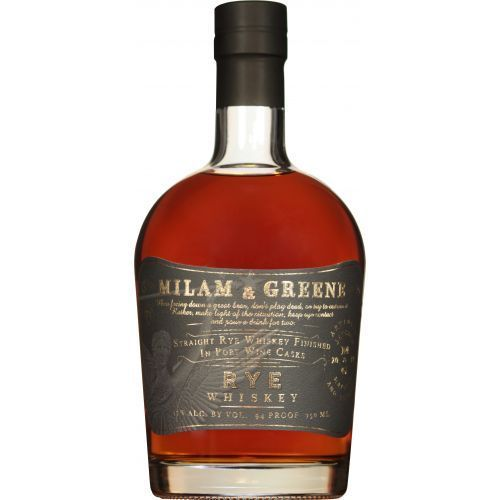Milam & Greene Port Finished Rye Whiskey is brought from Indiana to Texas where it is batched in the Blanco rickhouse and finished in old Port wine casks from Portugal. Some casks take longer than others, so the expert team tastes the whiskey finishing the in Port wine casks regularly to determine when optimal flavors are achieved.

 /* styles */ When: Friday, September 18, 6:00 p.m. CENTRAL Cost: Complimentary to participate! Register via Zoom link below.table div table+table+table+table+table+table+table+table+table+table+table+table+table+table+table+table+table+table div table{width:100%;padding:0}table div table+table+table+table+table+table+table+table+table+table+table+table+table+table+table+table+table+table div table img{width:96.23%;padding:0;float:none}table div table+table+table+table+table+table+table+table+table+table+table+table+table+table+table+table+table+table div table td{width:100%;padding:0 1.88% 18px}/* styles */YOUTUBE MANIA: SHALL WE WINE TV

 /* styles */ Did you know SWW had a YouTube Channel? Cool tips, tricks and insider scoop. Check it out! →
 table div table+table+table+table+table+table+table+table+table+table+table+table+table+table+table+table+table+table+table+table+table div table td,table.module-20{width:100%;padding:0}table div table+table+table+table+table+table+table+table+table+table+table+table+table+table+table+table+table+table+table+table+table div table{width:100%;float:none;margin-left:auto;margin-right:auto;padding:0}table div table+table+table+table+table+table+table+table+table+table+table+table+table+table+table+table+table+table+table+table+table div table a{border:0 none;text-decoration:none}table div table+table+table+table+table+table+table+table+table+table+table+table+table+table+table+table+table+table+table+table+table div table img{width:100%!important;border:0 none;text-decoration:none}/* styles */
 /* styles */
 /* styles */ To stay in the know with the latest and greatest. make sure to follow us all over social!/* styles */ CLICK BELOW
 table div table+table+table+table+table+table+table+table+table+table+table+table+table+table+table+table+table+table+table+table+table+table+table+table+table+table div table{width:100%;padding:0}table div table+table+table+table+table+table+table+table+table+table+table+table+table+table+table+table+table+table+table+table+table+table+table+table+table+table div table table{padding:0;float:left!important;width:26.059%!important}table div table+table+table+table+table+table+table+table+table+table+table+table+table+table+table+table+table+table+table+table+table+table+table+table+table+table div table td{padding-left:29px;padding-right:29px}table div table+table+table+table+table+table+table+table+table+table+table+table+table+table+table+table+table+table+table+table+table+table+table+table+table+table div table table+table,table div table+table+table+table+table+table+table+table+table+table+table+table+table+table+table+table+table+table+table+table+table+table+table+table+table+table div table table+table+table{float:left!important;width:26.059%!important}table div table+table+table+table+table+table+table+table+table+table+table+table+table+table+table+table+table+table+table+table+table+table+table+table+table+table div table table td,table div table+table+table+table+table+table+table+table+table+table+table+table+table+table+table+table+table+table+table+table+table+table+table+table+table+table div table table+table td,table div table+table+table+table+table+table+table+table+table+table+table+table+table+table+table+table+table+table+table+table+table+table+table+table+table+table div table table+table+table td{padding-left:0;padding-right:20px}table div table+table+table+table+table+table+table+table+table+table+table+table+table+table+table+table+table+table+table+table+table+table+table+table+table+table div table table+table+table+table{float:left!important;width:21.822999999999993%!important}table div table+table+table+table+table+table+table+table+table+table+table+table+table+table+table+table+table+table+table+table+table+table+table+table+table+table div table table+table+table+table td{padding-left:0;padding-right:0}/* styles */
 table div table+table+table+table+table+table+table+table+table+table+table+table+table+table+table+table+table+table+table+table+table+table+table+table+table+table+table div table{width:100%;padding:0}table div table+table+table+table+table+table+table+table+table+table+table+table+table+table+table+table+table+table+table+table+table+table+table+table+table+table+table div table img{width:96.23%;padding:0;float:none}table div table+table+table+table+table+table+table+table+table+table+table+table+table+table+table+table+table+table+table+table+table+table+table+table+table+table+table div table td{width:100%;padding:0 1.88% 18px}/* styles */SHALL WE WINE: THE MEMBERSHIP/* styles */ Shall We Wine Membership: The Ultimate Sip of Approval Host like a boss, dine like a boss… never miss a wine moment! Membership to Shall We Wine is our way of recognizing our most loyal wine tribe ⁠— the best way to access and take advantage of members-only perks. Shall We Wine membership is like one big soiree uniting beverage lovers far and wide. From novice to professional, we invite you to our society of fun! We offer 3 tiers of Shall We Wine membership: Cru (Community) Membership, Premier Cru Membership and ultimately, Grand Cru Membership. DETAILS COMING SOON!
 table div table+table+table+table+table+table+table+table+table+table+table+table+table+table+table+table+table+table+table+table+table+table+table+table+table+table+table+table+table+table+table div table{width:100%;padding:0}table div table+table+table+table+table+table+table+table+table+table+table+table+table+table+table+table+table+table+table+table+table+table+table+table+table+table+table+table+table+table+table div table img{width:96.23%;padding:0;float:none}table div table+table+table+table+table+table+table+table+table+table+table+table+table+table+table+table+table+table+table+table+table+table+table+table+table+table+table+table+table+table+table div table td{width:100%;padding:0 1.88% 18px}/* styles */REGISTER - Shall We Wine Educational Program w/Wanda Cole: Dessert Wines - 9/16/20 @ 6:00 PM CSTCOURSE DETAILS:
Dessert wines is perhaps the oldest style of wine. Pre-dating biblical times and modern fermentation techniques, dessert wines were made around the world from a magnitude of different grapes. From muscat, to grenache, to furmint these wines have been creating versatile, luscious styles that not only have a place at the dessert table but all throughout the meal complimenting a myriad of cooking styles and dishes. In this class we will examine major styles of dessert wines including production methods, historical relevance, geographical locations, grape varietals, sugar scales and wine assessment, serving techniques, and pairings.

A few highlights include Champagne, Tokaji, Sauternes, Sherry, Banyuls and Maury, Chenin Blanc, Riesling, and Ice Wine. Also included will be the various styles of muscat including Australian examples, Samos, Constantia, Moscato d'Asti, and Muscat d'Rivesaltes.

DISCOUNT OFFER FOR YOU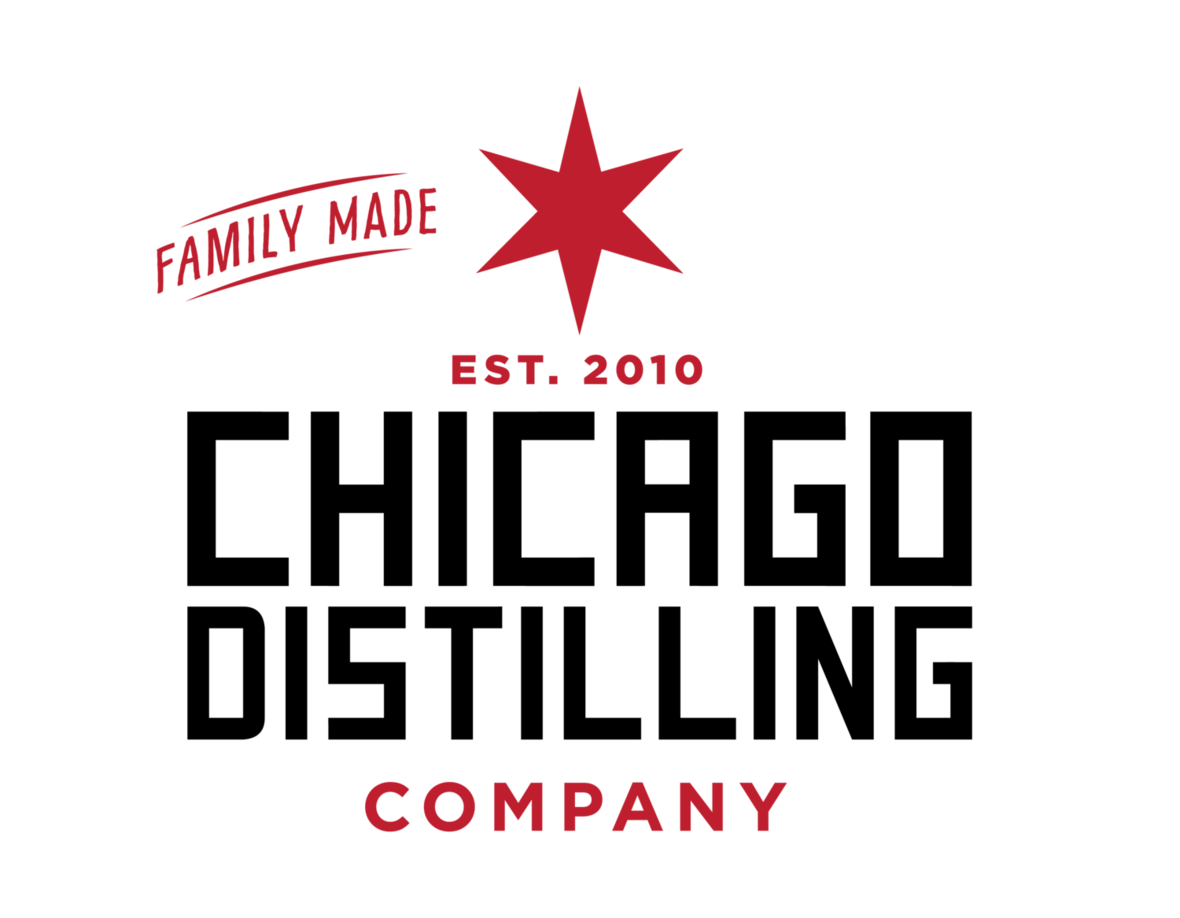Who doesn't love a discount?!

Our friends at *Chicago Distilling Company* are offering \$5 bottles of Finn's Gin. This gin and the owners were featured at our April 9, 2020 event.

Use code SWW5. This is for pickup only at their Chicago location.

 table div table+table+table+table+table+table+table+table+table+table+table+table+table+table+table+table+table+table+table+table+table+table+table+table+table+table+table+table+table+table+table+table+table+table+table+table div table{width:100%;padding:0}table div table+table+table+table+table+table+table+table+table+table+table+table+table+table+table+table+table+table+table+table+table+table+table+table+table+table+table+table+table+table+table+table+table+table+table+table div table img{width:96.23%;padding:0;float:none}table div table+table+table+table+table+table+table+table+table+table+table+table+table+table+table+table+table+table+table+table+table+table+table+table+table+table+table+table+table+table+table+table+table+table+table+table div table td{width:100%;padding:0 1.88% 18px}/* styles */SAVE THE DATE: UNITED WE TASTE9/19 - Regine will be a guest on a special segment of United We Taste by Cha Squared! 9AM Cali, 12PM NYC, 5PM London/Lisbon. Click here to check out the Cha Squared channel.

SAVE THE DATE: HOPE FOR HAITI

 /* styles */ 10/14 - Hope for Haitiand LS Cream Liqueur will be hosting an exciting virtual event! Every ticket sold will provide clean water to families and communities in Haiti.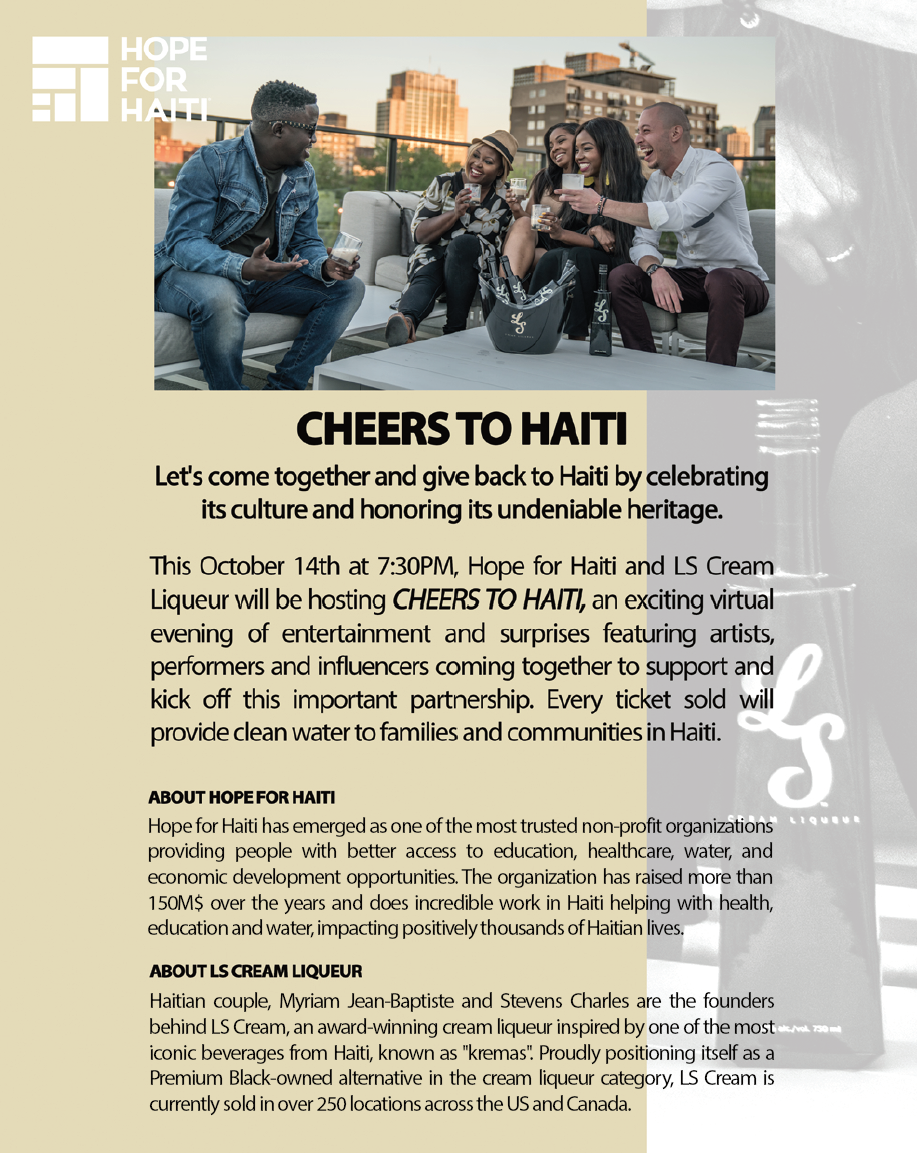table div table+table+table+table+table+table+table+table+table+table+table+table+table+table+table+table+table+table+table+table+table+table+table+table+table+table+table+table+table+table+table+table+table+table+table+table+table+table+table+table+table+table div table{width:100%;padding:0}table div table+table+table+table+table+table+table+table+table+table+table+table+table+table+table+table+table+table+table+table+table+table+table+table+table+table+table+table+table+table+table+table+table+table+table+table+table+table+table+table+table+table div table img{width:96.23%;padding:0;float:none}table div table+table+table+table+table+table+table+table+table+table+table+table+table+table+table+table+table+table+table+table+table+table+table+table+table+table+table+table+table+table+table+table+table+table+table+table+table+table+table+table+table+table div table td{width:100%;padding:0 1.88% 18px}/* styles */Wine Enthusiast's 2020 40 Under 40
Wine Enthusiast

Rising Black Voices In Wine
Wine & Spirits

 table div table+table+table+table+table+table+table+table+table+table+table+table+table+table+table+table+table+table+table+table+table+table+table+table+table+table+table+table+table+table+table+table+table+table+table+table+table+table+table+table+table+table+table+table+table div table{width:100%;padding:0}table div table+table+table+table+table+table+table+table+table+table+table+table+table+table+table+table+table+table+table+table+table+table+table+table+table+table+table+table+table+table+table+table+table+table+table+table+table+table+table+table+table+table+table+table+table div table img{width:96.23%;padding:0;float:none}table div table+table+table+table+table+table+table+table+table+table+table+table+table+table+table+table+table+table+table+table+table+table+table+table+table+table+table+table+table+table+table+table+table+table+table+table+table+table+table+table+table+table+table+table+table div table td{width:100%;padding:0 1.88% 18px}/* styles */It is incredibly moving to receive random notes of love! Below is from a fellow reader of Searching for Cloves & Lilies:

"Your book has blown me away. It is magnificent.. from the soul of you.. so deep and un self conscious. I got it this afternoon and read it from cover to cover. I need a second round to fully absorb the intensity. Mighty powerful! Thanks for printing and making available... not to mention creating!" Fondly, Lestable div table+table+table+table+table+table+table+table+table+table+table+table+table+table+table+table+table+table+table+table+table+table+table+table+table+table+table+table+table+table+table+table+table+table+table+table+table+table+table+table+table+table+table+table+table+table+table+table+table+table div table{width:100%;padding:0}table div table+table+table+table+table+table+table+table+table+table+table+table+table+table+table+table+table+table+table+table+table+table+table+table+table+table+table+table+table+table+table+table+table+table+table+table+table+table+table+table+table+table+table+table+table+table+table+table+table+table div table img{width:96.23%;padding:0;float:none}table div table+table+table+table+table+table+table+table+table+table+table+table+table+table+table+table+table+table+table+table+table+table+table+table+table+table+table+table+table+table+table+table+table+table+table+table+table+table+table+table+table+table+table+table+table+table+table+table+table+table div table td{width:100%;padding:0 1.88% 18px}/* styles */SWW CONCIERGE SERVICE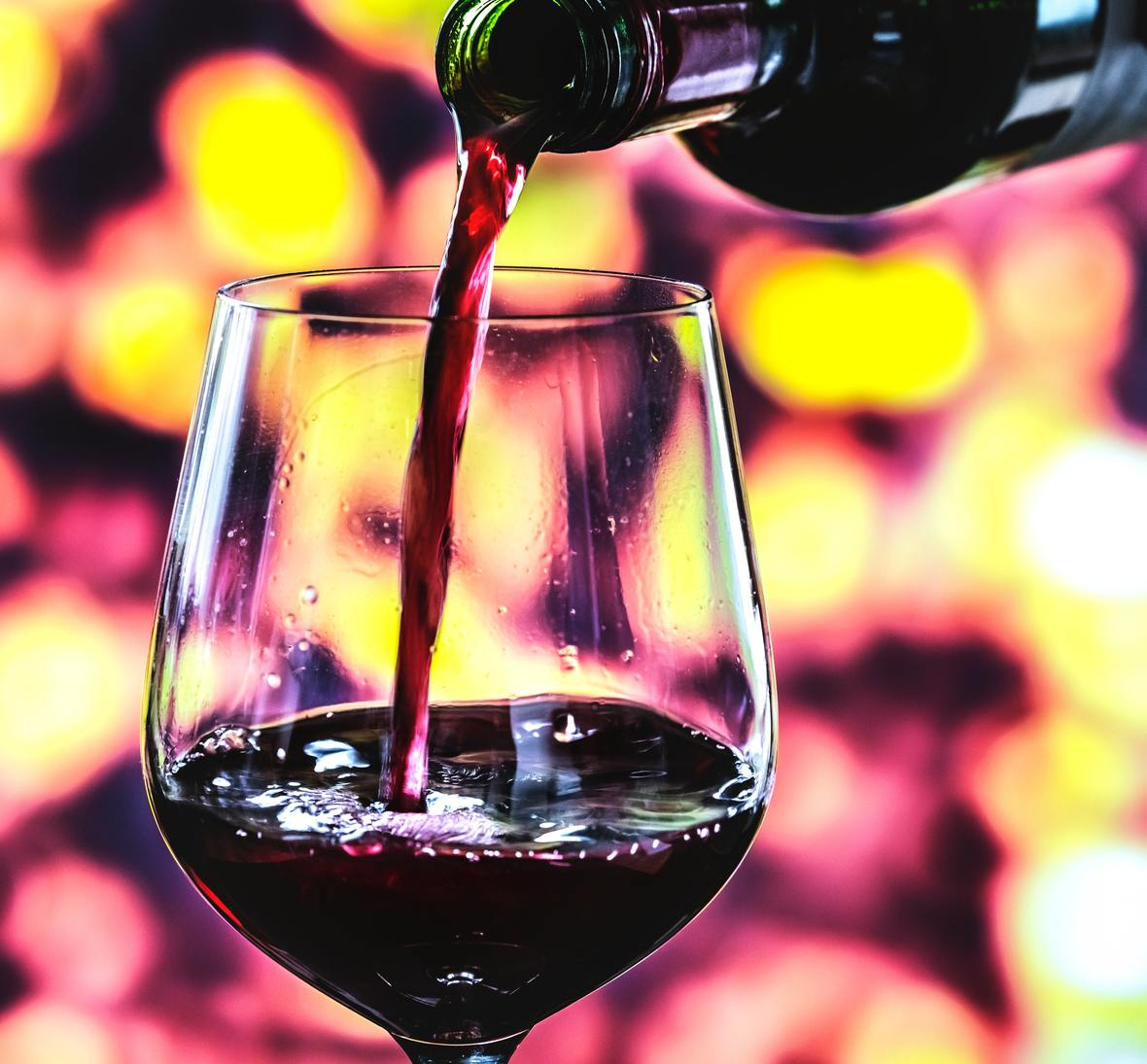Want a custom, tailor-made wine experience?

Sit down (virtually) with Shall We Wine for an exclusive Consultation and Personal Wine Profile! This small group event begins with a wine survey to determine your wine preferences. At the virtual event, we will discuss your wine style. Within a week we will generate a personalized list of 12 wines for you to explore.

 table div table+table+table+table+table+table+table+table+table+table+table+table+table+table+table+table+table+table+table+table+table+table+table+table+table+table+table+table+table+table+table+table+table+table+table+table+table+table+table+table+table+table+table+table+table+table+table+table+table+table+table+table+table+table div table{width:100%;padding:0}table div table+table+table+table+table+table+table+table+table+table+table+table+table+table+table+table+table+table+table+table+table+table+table+table+table+table+table+table+table+table+table+table+table+table+table+table+table+table+table+table+table+table+table+table+table+table+table+table+table+table+table+table+table+table div table img{width:96.23%;padding:0;float:none}table div table+table+table+table+table+table+table+table+table+table+table+table+table+table+table+table+table+table+table+table+table+table+table+table+table+table+table+table+table+table+table+table+table+table+table+table+table+table+table+table+table+table+table+table+table+table+table+table+table+table+table+table+table+table div table td{width:100%;padding:0 1.88% 18px}/* styles */DID YOU KNOW...We have a blog! Here you will find things like our Wine of the Week and other special wine & spirits topics! Stay in the know and read up on some of the cool things we've been sharing.

 table div table+table+table+table+table+table+table+table+table+table+table+table+table+table+table+table+table+table+table+table+table+table+table+table+table+table+table+table+table+table+table+table+table+table+table+table+table+table+table+table+table+table+table+table+table+table+table+table+table+table+table+table+table+table+table+table+table div table{width:100%;padding:0}table div table+table+table+table+table+table+table+table+table+table+table+table+table+table+table+table+table+table+table+table+table+table+table+table+table+table+table+table+table+table+table+table+table+table+table+table+table+table+table+table+table+table+table+table+table+table+table+table+table+table+table+table+table+table+table+table+table div table img{width:96.23%;padding:0;float:none}table div table+table+table+table+table+table+table+table+table+table+table+table+table+table+table+table+table+table+table+table+table+table+table+table+table+table+table+table+table+table+table+table+table+table+table+table+table+table+table+table+table+table+table+table+table+table+table+table+table+table+table+table+table+table+table+table+table div table td{width:100%;padding:0 1.88% 18px}/* styles */THANKS AND TOGETHERNESSIn an effort to maintain social distancing measures, Shall We Wine will continue to devote our time to connecting with each and every one of our friends and supporters like you virtually. We're constantly sharing fun content to help brighten up your weeks!

We appreciate your patience and cooperation as we work through these times together. Thank you for your continued support in making Shall We Wine your go-to wine & lifestyle resource!/* styles */ Learn, Drink, Repeat! The Shall We Wine Team
 Like   Tweet   Pin   +1   in